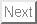Academic Paper
Kunichika Tsumoto, Tetsushi Ueta, Tetsuya Yoshinaga, Hiroshi Kawakami, Bifurcation analyses of nonlinear dynamical systems: From theory to numerical computations, Institute of Electronics, Information and Communication Engineers, Nonlinear Theory and Its Applications, IEICE, Vol.3, No.4, 458-476, Oct. 2012.
Abstract: In this paper, we explain how to compute bifurcation parameter values of periodic solutions for non-autonomous nonlinear differential equations. Although various approaches and tools are available for this problem nowadays, we introduce the simplest method composed by computational algorithms appeared in textbooks for beginner's, i.e., the Newton's method and Runge-Kutta method. We formulate the given bifurcation problem as a boundary value problem and use the Newton's method as a solver consistently. All derivatives required in each iteration are obtained by solving variational equations about the state and the parameter. Thank to quadratic convergence ability of the Newton's method, accurate results can be obtained without using any sophisticated mathematical library or software. If a discontinuous periodic force is applied to the system, we can take the same strategy to solve the bifurcation problem. The key point of this method is deriving a differentiable composite map from consideration of the various information about the problem such as the location of sections, the periodicity, the Poincaré mapping, and so on.

Contact address: ueta @ tokushima-u.ac.jp
2020-10-01